Next: 6.3 Unary relational operations Up: 6 Creating New Relations Previous: 6.1 Important warning

# 6.2 Binary relational operations

Relation Union(Relation &r, Relation &r)
Works for both relations and sets. The arguments must have the same arity. Ifandthen the result is.

Relation Intersection(Relation &r, Relation &r)
Works for both relations and sets. The arguments must have the same arity. If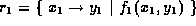andthen the result is.

Relation Composition(Relation &r, Relation &r)
Works for relations only. The output arity of rmust be same as the input arity of r. Ifandthen the result is.

Relation Join(Relation &r, Relation &r)
Equivalent to Composition(r, r) .

Relation Restrict_Domain(Relation &r, Relation &r)
First argument must be a relation and second argument must be a set. The input arity of rmust be the same as the arity of r. Ifandthen the result is.

Relation Restrict_Range(Relation &r, Relation &r)
First argument must be a relation and second argument must be a set. The output arity of rmust be the same as the arity of r. Ifandthen the result is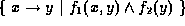.

Relation Difference(Relation &r, Relation &r)
Works for both relations and sets. The arguments must have the same arity. Ifandthen the result is.

Relation Cross_Product(Relation &r, Relation &r)
Works for sets only. Ifandthen the result is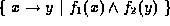.

Relation Gist(Relation &r, Relation &r, int effort=0)
Works for both relations and sets. The arguments must have the same arity. Ifand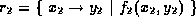then the result is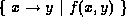where f is a presburger formula such thatNote: there are many f's that satisfy this property, so the results of this operation are not completely specified. We did this deliberately as our method for computing f may change in future releases. The effort parameter specifies how hard we try to make f tight, 0 represents least effort and 2 represents most effort.Next: 6.3 Unary relational operations Up: 6 Creating New Relations Previous: 6.1 Important warning

omega@cs.umd.edu

Web Accessibility